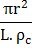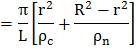# A copper wire of lengthand radiusis nickel plated till its final radius becomebut length remains. If the resistivity of nickel and copper beandrespectively, the conductance of the nickelled wire is a)b)c)d)## Question ID - 150322 :- A copper wire of lengthand radiusis nickel plated till its final radius becomebut length remains. If the resistivity of nickel and copper beandrespectively, the conductance of the nickelled wire is a)b)c)d)3537

 (c) Resistance of copper part of wireand Resistance of nickel portion of wireAs these two resistances are in parallel, hence conductance of the nickelled wireNext Question :

Following figure shows cross-sections through three long conductors of the same length and material, with square cross-section of edge lengths as shown. Conductorwill fit snugly within conductorand conductorwill fit snugly within conductorRelationship between their end to end resistance isa)b)c)d) Information is not sufficient